# Parallel Lines in a Quadrilateral II

### Problem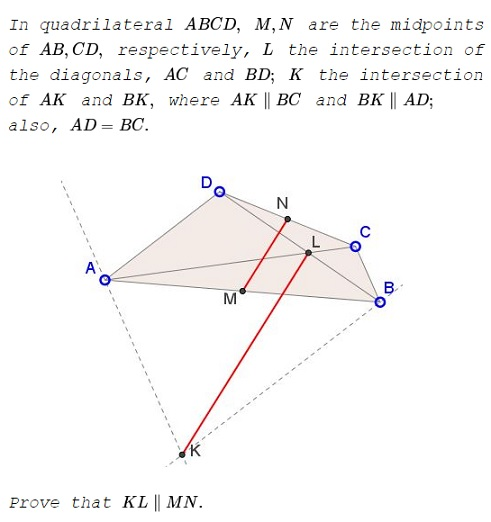### Solution 1

The constraint $AD=BC\,$ is not necessary. Let in barycentric coordinates, $D = (u: v: w),\,$ $M = (1: 1: 0),\,$ $N = (u: v: u + v + 2 w),\,$ $L = (u: 0: w),\,$ $K = (v + w: w: -w).$

The line $MN\,$ has equation

$MN:\;(u + v + 2 w) x + (-u - v - 2 w) y + (-u + v) z = 0.$

The line $KL\,$ has equation

$KL:\;w x + (-u - v - w) y - u z = 0.$

Both lines go through the infinite point

$(v + w: u + w: -u - v - 2 w).$

### Solution 2

Let $P\,$ be the intersection of $AD\,$ and $BC.\,$ Also, let $AD=BC=x,\,$ $PA=a\,$ and $PB=b;\,$ $w\,$ the internal bisector at $P\,$ of $\Delta APB.$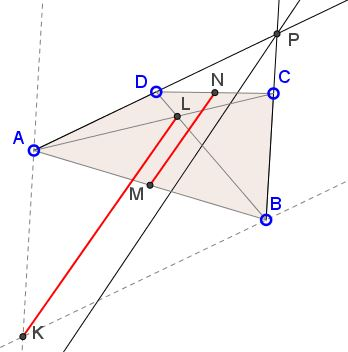This is a well known result (called teorema bisectoarei glisante in Romanian literature) that $AD=BC\,$ implies $w\parallel MN.$

Denote the areas $[PAL]=m,\,$ $[PBL]=n,\,$ $[ABL]=p.\,$ Obviously, $\displaystyle\overrightarrow{PL}=\frac{n\overrightarrow{PA}+m\overrightarrow{PB}}{m+n+p}.\,$ We have

$\displaystyle\frac{m}{n}+1+\frac{p}{n}=\frac{b-x}{a-x}+1+\frac{x}{a-x}=\frac{a+b-x}{a-x}.$

It follows that $\displaystyle\frac{n}{m+n+p}=\frac{a-x}{a+b-x}\,$ and, similarly, $\displaystyle\frac{m}{m+n+p}=\frac{b-x}{a+b-x}.\,$ Thus,

$\displaystyle\overrightarrow{PL}=\frac{(a-x)\overrightarrow{PA}+(b-x)\overrightarrow{PB}}{a+b-x}.$

But $PAKB\,$ is a parallelogram, such that $\overrightarrow{PK}=\overrightarrow{PA}+\overrightarrow{PB}\,$ so that

$\displaystyle\overrightarrow{LK}=\overrightarrow{PK}-\overrightarrow{PL}=\frac{b\overrightarrow{PA}+a\overrightarrow{PB}}{a+b-x}.$

However, observe that, from the Angle Bisector theorem, the direction $w\,$ is $b\overrightarrow{PA}+a\overrightarrow{PB},\;$ implying $LK\parallel w.$

### Solution 3

Let $S\,$ be a point on $AK\,$ such that $BS\parallel AC:$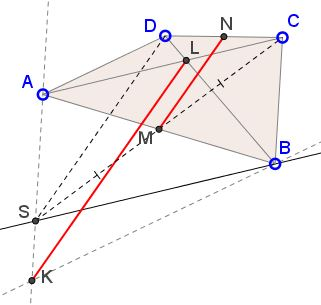It follows that $M\,$ is the of $CS\,$ so that $MN\parallel DS\,$ as the midline in $\Delta CDS.$

Further, $AD\parallel KB,\,$ $AL\parallel BS,\,$ implying that $SD\parallel KL.\,$ By transitivity, $KL\parallel MN.$

### Solution 4

Let $E=DM\cap BK,\,$ then $MD=ME\,$ and $AD=BE.\,$ In $\Delta DEC,$ $MN\parallel CE,\,$ as a midline.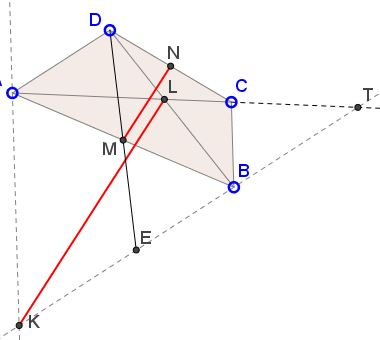Let $T=AC\cap BK.\,$ Then $BC\parallel AK,\,$ so that, by Thales' Theorem, $\displaystyle\frac{CT}{AT}=\frac{BT}{KT}.\,$ Further, since $AD\parallel BT,\,$ $\displaystyle\frac{LT}{AL}=\frac{BT}{AD}\,$ and, subsequently, we obtain a series of implied proportions:

$\displaystyle\frac{LT}{LT+AL}=\frac{BT}{BT+AD},\,$ $\displaystyle\frac{LT}{AT}=\frac{BT}{BT+BE},\,$ $\displaystyle\frac{LT}{AT}=\frac{BT}{ET}.$

Putting everything together shows that

$\displaystyle\frac{\displaystyle\frac{CT}{AT}}{\displaystyle\frac{LT}{AT}}=\frac{\displaystyle\frac{BT}{KT}}{\displaystyle\frac{BT}{ET}}\,$

and, thus, $\displaystyle\frac{CT}{LT}=\frac{ET}{KT},\,$ from which, by the inverse of Thales' theorem, $KL\parallel MN.$

### Solution 5

Lemma 1

With a reference to the diagram below, if $M',N'\,$ are he midpoints, $A'D'=B'C',\,$ then $M'N'\,$ is parallel to the bisector of $\angle A'E'B'.$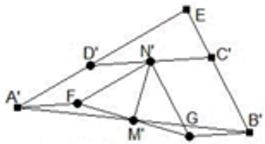Construct parallelogram $A'F'N'D'\,$ and $N'GB'C';\,$ triangle $FGN'\,$ is isosceles, $A'F\parallel B'G\,$ and the two are equal, so $M'\,$ is the midpoint of $FG,\,$ the base of the isosceles triangle $FGN',\,$ so the median $N'M'\,$ is also the bisector of $\angle FN'G,\,$ which has $FN'\parallel EA'\,$ and $N'G\parallel EB'.$

Lemma 2

With a reference to the diagram below, if $AD=BC,\,$ $L\,$ the intersection of $AC\,$ and $BD,\,$ $BK\parallel AD,\,$ $AK\parallel BC,\,$ then $KL\\,$ is the bisector of $\angle AKB.$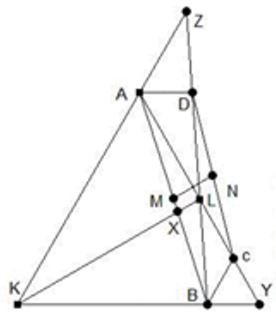Let $X\,$ be the intersection of $AB\,$ and $KL,\,$ $Y\,$ that of $KB\,$ and $AC,\,$ $Z\,$ the intersection of $AK\,$ and $BD.\,$ Then $\displaystyle\frac{AZ}{KZ}=\frac{AD}{KB},\,$ $\displaystyle\frac{KY}{BY}=\frac{AK}{BC}.\,$ By the converse of Ceva's Theorem for $\Delta AKB,\,$ with the concurrence at $L,\,$ $\displaystyle\frac{AZ}{KZ}\cdot\frac{KY}{BY}\cdot\frac{BX}{AX}=1;\,$ with the previous equalities we obtain $\displaystyle\frac{AX}{BX}=\frac{AK}{BK},\,$ making $KL\,$ the angle bisector of $\angle AKB.$

### Solution 6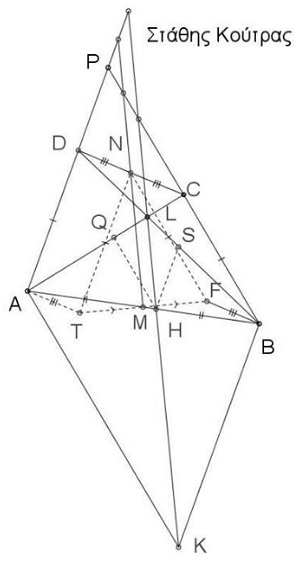$\Delta NTF\,$ is isosceles and $MN\,$ is a median, therefore, it is also the angle bisector of $\angle TNF.\,$ Thus $MN\,$ is parallel to the angle bisector at $P\,(1).\,$ Draw $HQ\parallel BC\,$ and $HS\parallel AD.\,$ We have $HA:AB=HQ:BC\,(2)\,$ and $HB:AB=HS:AD.\,(3)\,$ From $(2),(3)\,$ $HA:HB=HQ:HS\,(4).\,$ $HQ:KA=HS:KB\,(=LH:LK)\,$ so that $HQ:HS=KA:KB\,(5).\,$ From $(4),(5),\,$ $KA:KB=HA:HB,\,$ implying that $KL\,$ is angle bisector of the angle at $K\,(6).\,$ Thus, $PAKB\,$ is a parallelogram $(7).\,$ Now $(1),(6),(7)\,$ imply that $MN\parallel KL.$

### Solution 7

Without using $AD=BC,\,$ let $P\,$ be the intersections of $AD,BC\,$ and $R\,$ be the midpoint of $PL.\,$ Then:

1. $R,M,N\,$ collinear (Gauss-Newton line).
2. $M\,$ belongs to $KP,\,$ since $KAPB\,$ parallelogram!
3. In triangle $PLK,\,$ $RM\,$ connects two midpoints, hence $RM\parallel KL.\,$ QED.

### Acknowledgment

The problem has been kindly posted by Leo Guigiuc at the CutTheKnotMath facebook page. The problem, due to Stathis Koutras, has been originally posted at the Οι Ρομαντικοι της Γεωμετριας (Romantics of Geometry) facebook group. Solution 1 is by Francisco Javier García Capitán; Solution 2 is by Leo Giugiuc; Solution 3 is by a Vietnamese mathematician who signs up in Kanji; Solution 4 is by Stathis Koutras; Solution 5 is by Stan Fulger; Solution 6 is by Vaggelis Stamatiadis; Solution 7 by Dimitris Papadimitriou.

Note there is another set of parallel lines in a quadrilateral.